Courses

# Technical Test SSC JE: Electrical Engineering (EE)- 13

## 100 Questions MCQ Test Electrical Engineering SSC JE (Technical) | Technical Test SSC JE: Electrical Engineering (EE)- 13

Description
This mock test of Technical Test SSC JE: Electrical Engineering (EE)- 13 for Electrical Engineering (EE) helps you for every Electrical Engineering (EE) entrance exam. This contains 100 Multiple Choice Questions for Electrical Engineering (EE) Technical Test SSC JE: Electrical Engineering (EE)- 13 (mcq) to study with solutions a complete question bank. The solved questions answers in this Technical Test SSC JE: Electrical Engineering (EE)- 13 quiz give you a good mix of easy questions and tough questions. Electrical Engineering (EE) students definitely take this Technical Test SSC JE: Electrical Engineering (EE)- 13 exercise for a better result in the exam. You can find other Technical Test SSC JE: Electrical Engineering (EE)- 13 extra questions, long questions & short questions for Electrical Engineering (EE) on EduRev as well by searching above.
QUESTION: 1

Solution:
QUESTION: 2

Solution:
QUESTION: 3

### Consider the circuit as shown below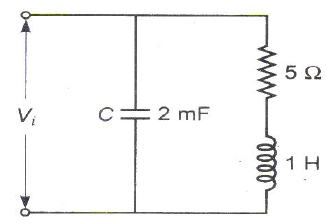The Q factor of the inductor is equal to

Solution:
QUESTION: 4

If a sinusoidal voltage source is connected to a power transformer, its no load current would be

Solution:
QUESTION: 5

Short-circuit test is performed on a transformer with a certain impressed voltage at rated frequency. If the short-circuit test is now performed with the same magnitude of impressed voltage but at a frequency higher than the rated frequency, then

Solution:
QUESTION: 6

If a 230 V DC series motor is connected to a 230 V AC supply, then the motor will

Solution:
QUESTION: 7

A pair of similar DC shunt generators operate in parallel and supply a common load. It is
required to switch-off machine 1 and allow machine 2 to supply the entire load. The
following operations are to be used to achieve this
1. Switch-off the main switch of machine 1.
2. Reduce the field current of machine 1.
3. Increase the field current of machine 2.
4. Ensure that machine 1 just floats.
The correct sequence of these operations is

Solution:
QUESTION: 8

Match List-I with List-II and select the correct answer using the codes given below the lists.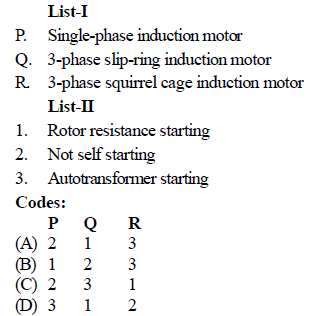Solution:
QUESTION: 9

As compared to DOL starting, a cage induction motor with star-delta starting shall have

Solution:
QUESTION: 10

The speed of a 3-phase induction motor operating in its stable region

Solution:
QUESTION: 11

Match List-I (power factor) with List-II (armature reaction of an alternator) and select
the correct answer using the codes given below the lists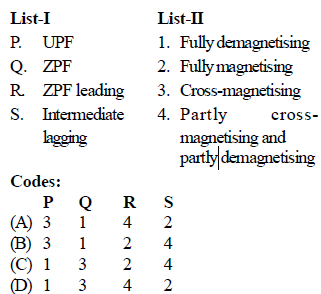Solution:
QUESTION: 12

While a 2-pole AC servomotor is in operation, if the voltage across the control field winding becomes zero, then the motor has a tendency to run as a single-phase induction motor. To prevent this

Solution:
QUESTION: 13

Which of the following single-phase motor has highest starting torque

Solution:
QUESTION: 14

If an AC servomotor has one of its winding excited by AC, then voltage measured at the other winding with rotor running will be

Solution:
QUESTION: 15

On which one of the following cycles does a modern steam plant work

Solution:
QUESTION: 16

The most appropriate operating speeds in rpm of generator used in thermal, nuclear and hydropower plants would be

Solution:
QUESTION: 17

In the network as shown in figure the marked parameters are per unit impedances. The busadmittance matrix of the network is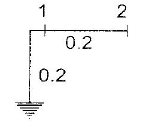Solution:
QUESTION: 18

The Buchholz relay protects a transformer from

Solution:
QUESTION: 19

If the fault current is 3000 A for a relay with a plug setting of 50% and CT ratio of 1000 : 1,the plug setting multiplier would be

Solution:
QUESTION: 20

Maloperation of differential protection of transformers due to magnetizing in rush current is prevented by

Solution:
QUESTION: 21

In twBo wattmeter method of measuring 3-phase power, power factor is 0.5, then one of the wattmeter will read

Solution:
QUESTION: 22

Schering bridge is used to

Solution:
QUESTION: 23

Following figures show four bridges. Which figure is Owen's bridge

Solution:
QUESTION: 24

The power in a resistor R is estimated measuring the voltage and current using voltmeter-ammeter method. Two different arrangements can be used as shown in circuit I and II. Less erroneous results are obtained adapting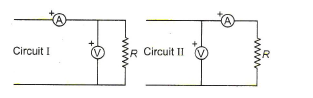Solution:
QUESTION: 25

A CRO uses

Solution:
QUESTION: 26

The latching current of SCR is 18 mA. Its holding current will be

Solution:
QUESTION: 27

The maximum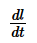in a SCR is

Solution:
QUESTION: 28

In the figure below the, current of 1A flows through the resistance of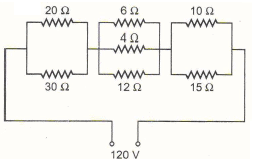Solution:
QUESTION: 29

Find the value of Vx in the given figure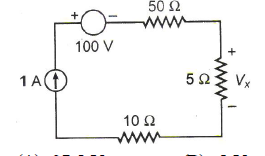Solution:
QUESTION: 30

Which of the following is correct regarding autotransformer

Solution:
QUESTION: 31

In a three-phase D/Y transformer shown in the figure, the phase displacement of secondary line voltage with corresponding primary line voltages will be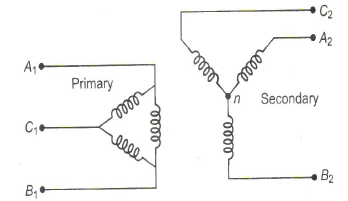Solution:
QUESTION: 32

If the speed of a DC motor increases with load torque, then it is a

Solution:
QUESTION: 33

A 3-phase slip-ring induction motor is wound for 4-poles on stator and 6-poles on rotor.When 3-phase balanced voltage source at 50 Hz is applied to the motor, it will run at

Solution:
QUESTION: 34

The phasor diagram of a synchronous machine connected to an infinite bus is shown in the figure. The machine is acting as a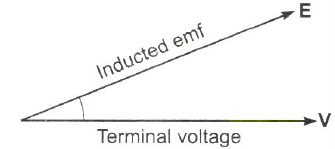Solution:
QUESTION: 35

Match List-I with List-II and select the correct answer using the codes given below the lists.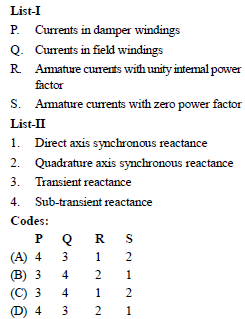Solution:
QUESTION: 36

Which of the following capacitors start splitphase induction motors will have the largest value of capacitance

Solution:
QUESTION: 37

An AC servomotor exhibits

Solution:
QUESTION: 38

In hydro power stations, what is an enlarged body of water just above the in take and used as a regulating reservoir

Solution:
QUESTION: 39

Resistance switching is normally resorted in case of

Solution:
QUESTION: 40

A Mho relay is a

Solution:
QUESTION: 41

Match List-I (Relays) with List-II (Protection) and select the correct answer using the codes
given below the lists: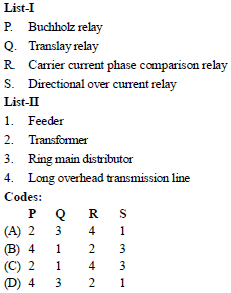Solution:
QUESTION: 42

The limiting errors of measurement of power consumed by and the voltage error resistanceare + 2% and + 3.5% respectively. The limiting error of measurement of resistance will be

Solution:
QUESTION: 43

In a gravity controlled instrument, the deflection angle is proportional to

Solution:
QUESTION: 44

The problem of electro-static coupling in a transformer is acute at

Solution:
QUESTION: 45

For power measurement of three-phase circuit by two wattmeter method, when the value of power factor is less than 0.5 lagging

Solution:
QUESTION: 46

Which bridge is used to determine frequency

Solution:
QUESTION: 47

Match List-I with List-II and select the correct answer using the codes given below the lists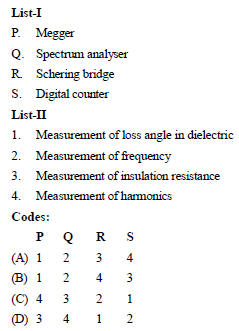Solution:
QUESTION: 48

In the Owen's bridge shown in below figure,Z1 = 200 Ð 60º, Z2 = 400 Ð – 90º, Z3 = 300Ð 0º,Z4 = 400 Ð 30º. Then, choose the correct statement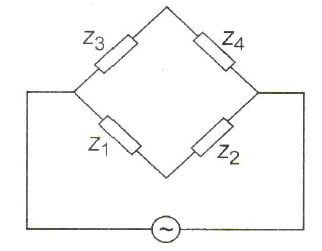Solution:
QUESTION: 49

Match List-I (Speed/torque regime in a DC motor) with List-II (The control methods
suitable for the same) and select the correct answer using the codes given below the lists.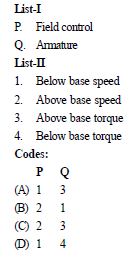Solution:
QUESTION: 50

In the circuit shown below, diodes have cut-in voltage of 0.6 V. The diode in On state is/are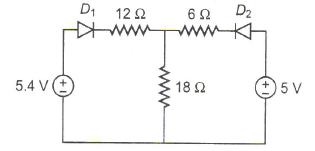Solution:
QUESTION: 51

In the circuit of the figure, the value of the voltage source E is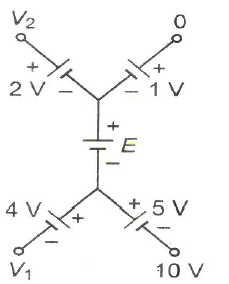Solution:
QUESTION: 52

In a nuclear power station using boiling water reactor, water is used as

Solution:
QUESTION: 53

In a DC transmission line

Solution:
QUESTION: 54

A heater connected to 230 V main supply draws a current of 7.5 A, then what is the
resistance of heating element

Solution:
QUESTION: 55

Find the equivalent resistance between the nodes P and Q in both of the different cases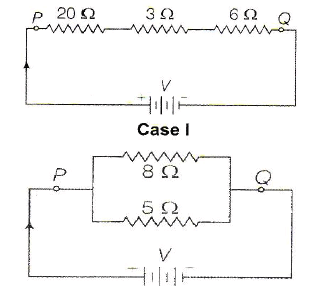Solution:
QUESTION: 56

The resistance of eureka wire is 2.5 W. What is the value of specific resistance of wire of 14m length and diameter of 0.14 cm

Solution:
QUESTION: 57

In the figure, a carbon resistor has band of different colours on its body. The resistance of the following body is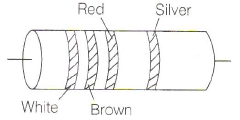Solution:
QUESTION: 58

A current of 16A is distributed in a circuit having two branches when connected on 220 V supply. If the resistance of one branch is 20W,then find the resistance of other branch and power taken by both the branches.

Solution:
QUESTION: 59

Determine the electrical energy of a circuit, if the current flowing from the source is 5A for duration of 2s and the resistance of the circuit is 5W

Solution:
QUESTION: 60

In which of the following, it is not desired to attain the condition of maximum power transfer

Solution:
QUESTION: 61

For which type of the following Thevenin's and Norton's equivalents cannot be developed

Solution:
QUESTION: 62

Two two-port networks are connected in cascade. The combination is to represent as a single two-port networks. The parameters of the network are obtained by multiplying the individual

Solution:
QUESTION: 63

Consider the following statements
1. Interpole windings are connected in series with armature winding
2. Polarity of interpole must be the same as that of the main pole in advance
3. Distortion of the main field under the pole shoes is not affected by the use of interpoles.

Of these statements regarding inter pole used in DC motors, the correct statement(s) is/are

Solution:
QUESTION: 64

'Crawling' in an induction motor is due to

Solution:
QUESTION: 65

Match List-I (type of single-phase motor) with List-II (type of appliance) and select the correct answer using the codes given below the lists.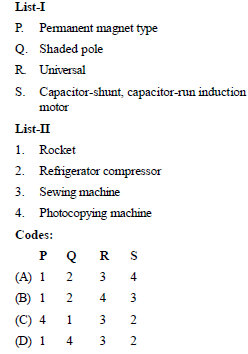Solution:
QUESTION: 66

A capacitor-start single-phase induction motor is switched-on to supply with its capacitor replaced by an inductor of equivalent reactance value. It will

Solution:
QUESTION: 67

Match List-I (Classification of head) with List- II (Types of turbine) and select the correct
answer using the codes given below the lists.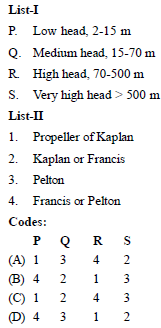Solution:
QUESTION: 68

In a nuclear power station using boiling water reactor, water is used as

Solution:
QUESTION: 69

A thermal power plant has installed capacity of 15000 MW. The annual load factor is 60% and capacity factor is 50%. The reverse capacity of the plant will be

Solution:
QUESTION: 70

If X is the system reactance and R is the resistance, the power transferred is maximum when

Solution:
QUESTION: 71

Match List-I (Types of relays) with List-II (Types of protection) and select the correct
answer using the codes given below the lists.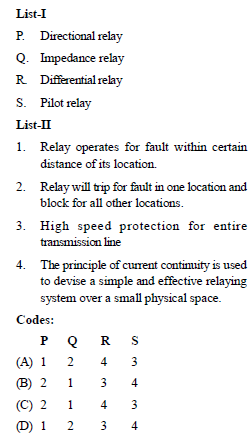Solution:
QUESTION: 72

The CT supplies current to the current coil of a wattmeter power factor meter, energy meter and an ammeter. These are connected as

Solution:
QUESTION: 73

Wien’s bridge is useful for measuring

Solution:
QUESTION: 74

X and Y plates of a CRO are connected to unequal voltages of equal frequency with phase shift of 90°. The Lissajaus figure on the screen will be

Solution:
QUESTION: 75

R1 and R4 are the opposite arms of a Wheat stone bridge as are R3 and R2. The source voltage is applied across R1 and R3. Under balanced conditions which one of the following is true?

Solution:
QUESTION: 76

A series circuit has two pure elements with entire network voltage and current equation as

V= 10 sin (l00 t + 80°) VI= 5 cos (l00 t + 50°)

A Then the two elements in the network will be

Solution:
QUESTION: 77

Consider the following statements in respect of important features of IGBT
1. It has a high impedance gate as that of a MOSFET.
2. It has a low on state voltage as that of a BJT.
3. It can block negative voltages as in the case of GTO.
Which of these statements are correct?

Solution:
QUESTION: 78

In the figure shown if we connect 2 V with internal resistance 1 Ω at AA’ with positive terminal at A’, the current through R in ampere is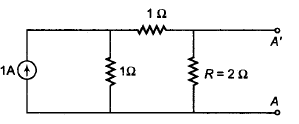Solution:
QUESTION: 79

Find voltmeter reading of the circuit shown below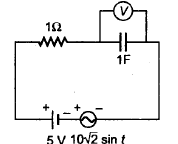Solution:
QUESTION: 80

The power supplied to the circuit in figure, by the voltage and current sources respectively are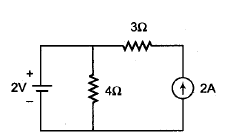Solution:
QUESTION: 81

If a fixed amount of power is to be transmitted over a certain length with fixed power loss, it can be said that volume of conductor is

Solution:
QUESTION: 82

Consider the following network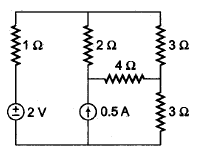The power dissipated across 4 Ω resistor is

Solution:
QUESTION: 83

The component of excitation current present in Δ-connected secondary of transformer are

Solution:
QUESTION: 84

In a loaded DC motor, if the bushes are given a shift from the inter polar axis in the direction of relation, then the communication will

Solution:
QUESTION: 85

The time constant for the given circuit in second is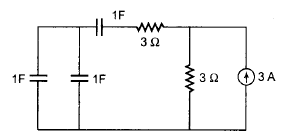Solution:
QUESTION: 86

In a thyristor, which of the following is correct?

Solution:
QUESTION: 87

Once SCR starts conducting forward current, its gate losses control over

Solution:
QUESTION: 88

For parallel operation of transformers, which  condition is essential?
1. Same voltage ratio.
2. Same pu leakage impedance.
3. Same Xe|re ratio.
4. Same polarity.

Solution:
QUESTION: 89

Match List-I with List-II and select the correct answer using the codes given below the lists.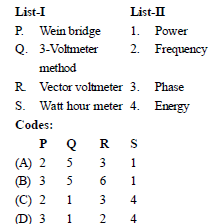Solution:
QUESTION: 90

A galvanometer of resistance 15 Ω gives full scale deflection for a current of 2 mA. Calculate the shunt resistance needed to convert it to an ammeter of range 0 to 5 A.

Solution:
QUESTION: 91

A moving coil galvanometer has a resistance of 25 Ω and gives a full scale deflection for a currentof 10 mA. How will you convert it into voltmeter having range 0–100 V?

Solution:
QUESTION: 92

Two transformers of different kVA ratings working in parallel, share the load in proportionto their ratings when their

Solution:
QUESTION: 93

In a transformer zero voltage regulation at fullload is

Solution:
QUESTION: 94

The torque speed characteristic of a repulsion motor resembles which of the following DC motor characteristic

Solution:
QUESTION: 95

A D/D connected transformer is connected to V/V connected transformer. The ratio of VA rating of V/V connected transformer and D/D connected transformer is

Solution:
QUESTION: 96

Consider the following statements:

The armature torque in a DC motor is a function of
1. Field flux
2. Armature current
3. Speed
4. Damping
Which of the above statements is/are correct

Solution:
QUESTION: 97

Insulating materials have the function of

Solution:

The most important function of the insulating material is to insulate voltage-carrying conductors against one another as well as against earth. But, in addition, they must fre- quently perform mechanical functions and must be in a position to withstand certain thermal and chemical stresses.

QUESTION: 98

A 50 Hz, 3-phase synchronous generator has inductance per phase of 15 mH. The capacitance of generator and circuit beaker is 0.002 mF. What is its natural frequency of oscillation

Solution:
QUESTION: 99

Relative permeability of iron is 4000. What is its magnetic susceptibility

Solution:
QUESTION: 100

(i) The peak voltage of an AC supply is 300V. What is the rms voltage
(ii) The rms value of current in an AC circuit is 10A. What is the peak current

Solution: